Most Affordable JEE | NEET | 8,9,10 Preparation by Kota's Top IITian Doctor Faculties

# NCERT Solutions for Class 9 Maths chapter 5 Exercise 5.1 - Introduction to Euclid's geometry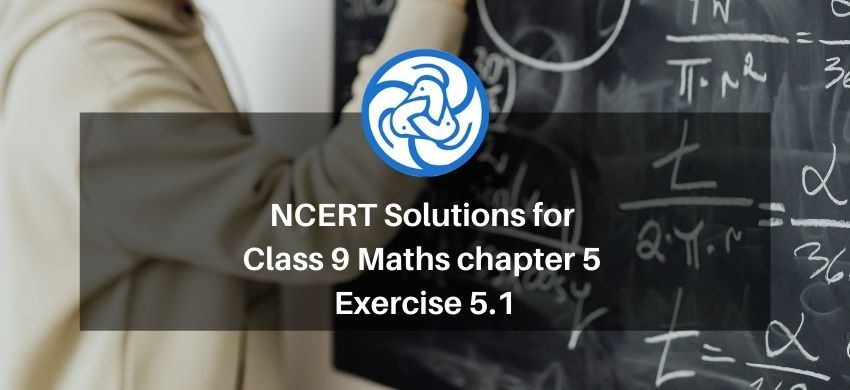`
Hey, are you a class 9 Student and Looking for Ways to Download NCERT Solutions for Class 9 Maths chapter 5 Exercise 5.1? If Yes then you are at the right place.

Here we have listed Class 9 maths chapter 5 exercise 5.1 solutions in PDF that is prepared by Kota’s top IITian’s Faculties by keeping Simplicity in mind.

If you want to score high in your class 9 Maths Exam then it is very important for you to have a good knowledge of all the important topics, so to learn and practice those topics you can use eSaral NCERT Solutions.

So, without wasting more time Let’s start.

### Download The PDF of NCERT Solutions for Class 9 Maths chapter 5 Exercise 5.1 "Introduction to Euclid's geometry"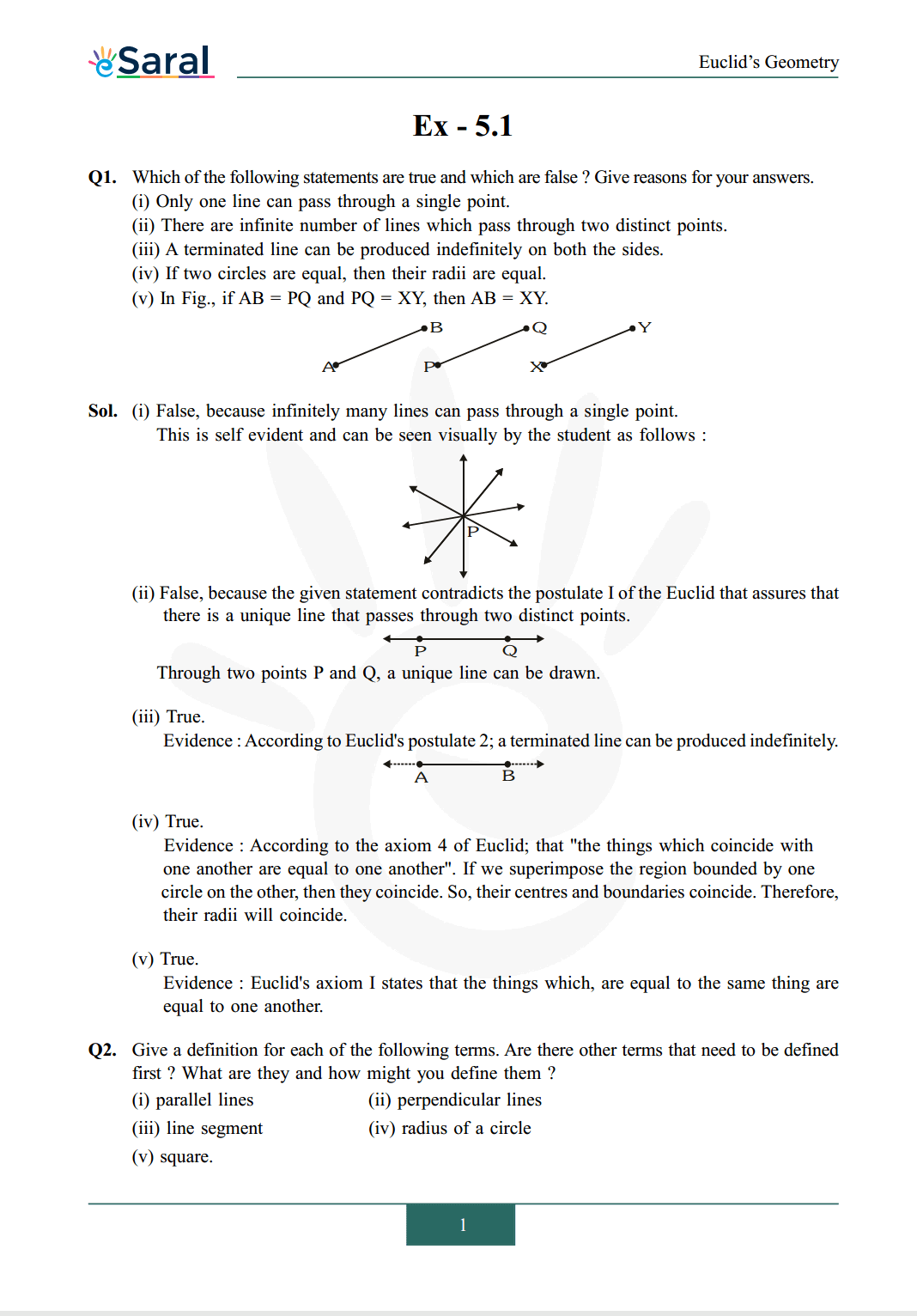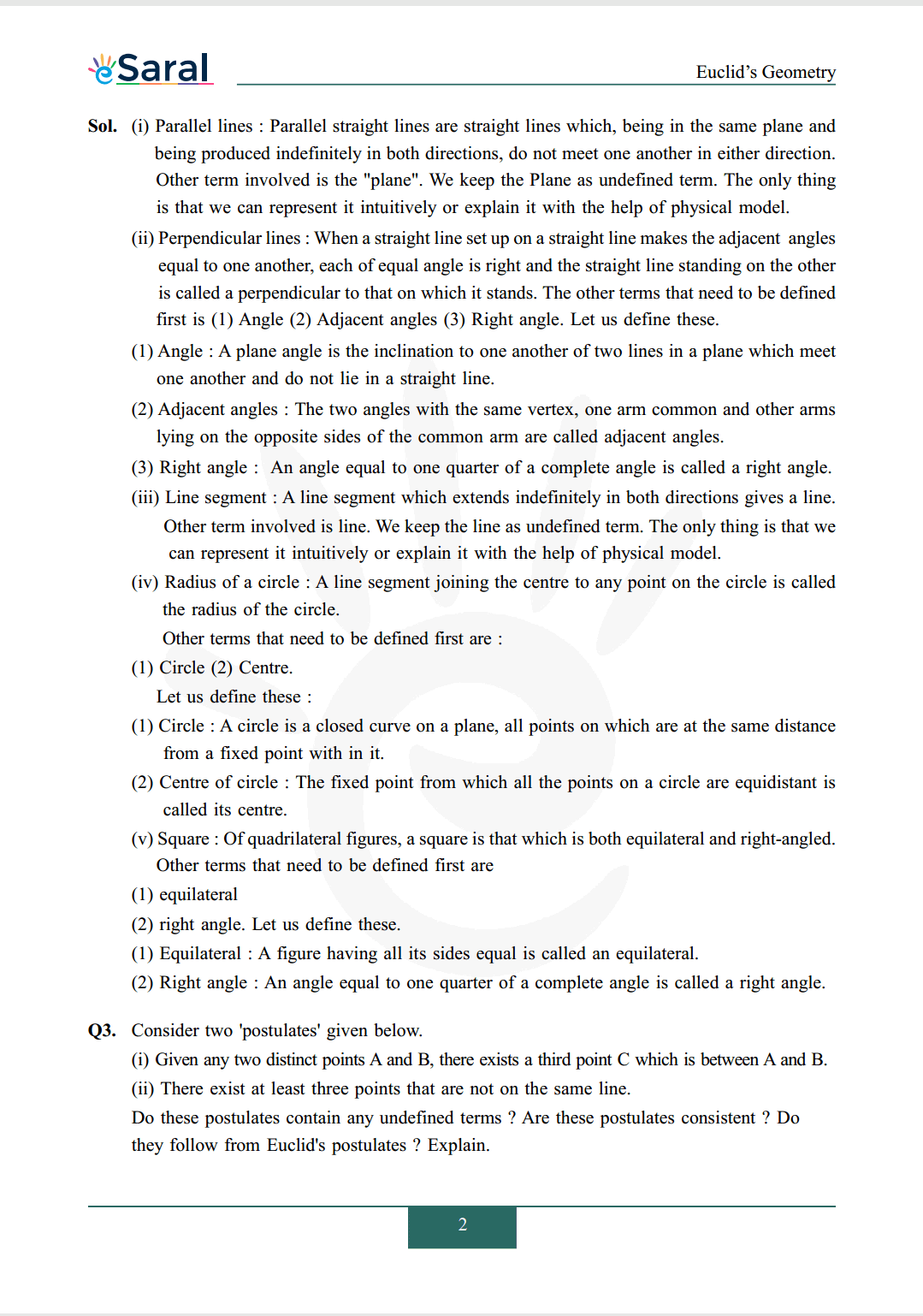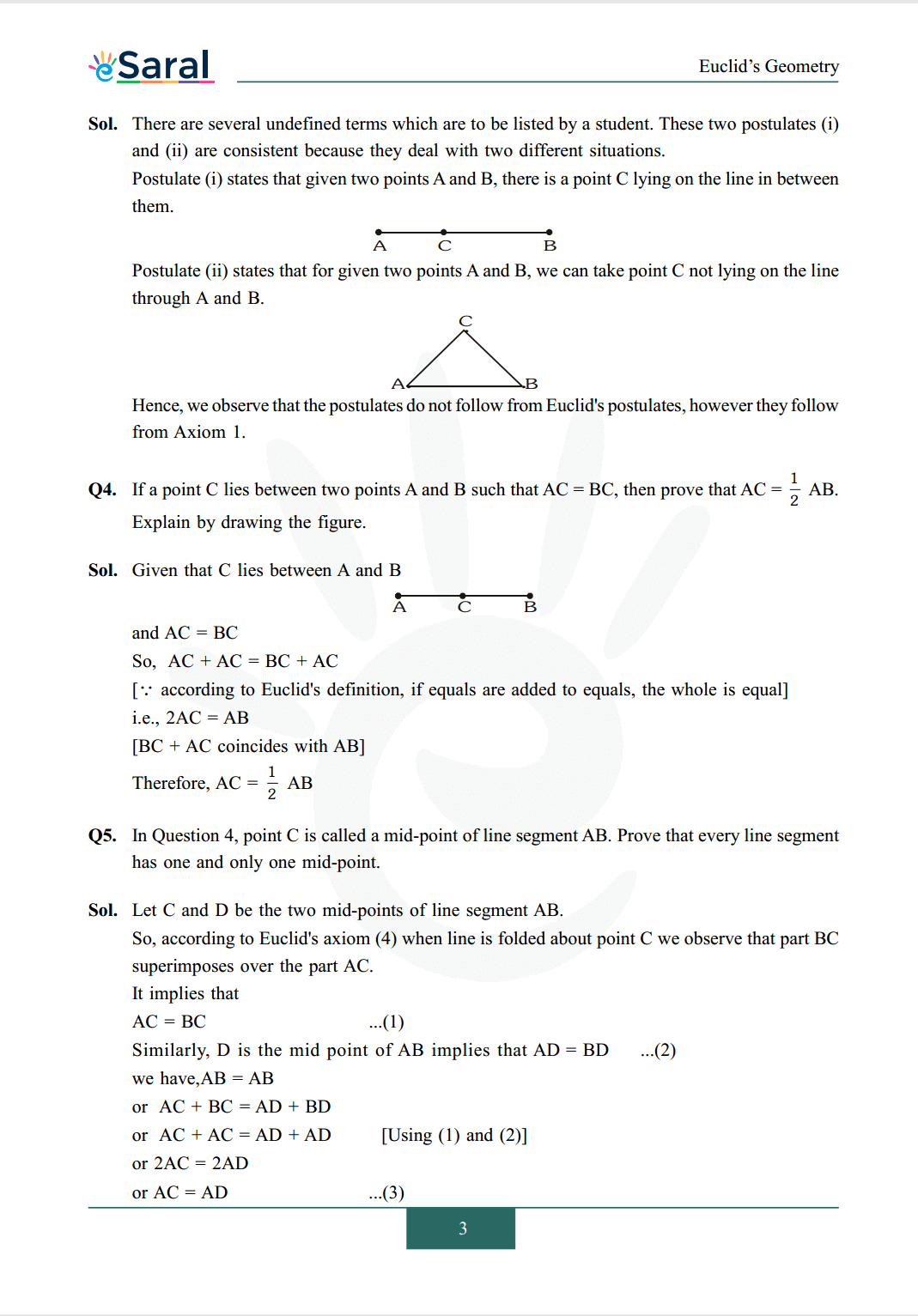#### All Questions of Chapter 5 Exercise 5.1

Once you complete the chapter 5 then you can revise Ex. 5.1 by solving following questions

Q1. Which of the following statements are true and which are false? Give reasons for your answers.
(i) Only one line can pass through a single point.
(ii) There are infinite number of lines which pass through two distinct points.
(iii) A terminated line can be produced indefinitely on both the sides.
(iv) If two circles are equal, then their radii are equal.
(v) In Fig., if $\mathrm{AB}=\mathrm{PQ}$ and $\mathrm{PQ}=\mathrm{XY}$, then $\mathrm{AB}=\mathrm{XY}$.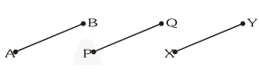Q2. Give a definition for each of the following terms. Are there other terms that need to be defined first? What are they and how might you define them?
(i) parallel lines
(ii) perpendicular lines
(iii) line segment
(v) square.